# Learning C++ Functional Programming3.3 (4 reviews total)
By Wisnu Anggoro
• FREE Subscription Access now
• \$10.00 Was 39.99 eBook
What do you get with a Packt Subscription?

• Constantly updated with 100+ new titles each month
• Breadth and depth in over 1,000+ technologies

Functional programming allows developers to divide programs into smaller, reusable components that ease the creation, testing, and maintenance of software as a whole. Combined with the power of C++, you can develop robust and scalable applications that fulfill modern day software requirements. This book will help you discover all the C++ 17 features that can be applied to build software in a functional way.The book is divided into three modules—the first introduces the fundamentals of functional programming and how it is supported by modern C++. The second module explains how to efficiently implement C++ features such as pure functions and immutable states to build robust applications. The last module describes how to achieve concurrency and apply design patterns to enhance your application’s performance. Here, you will also learn to optimize code using metaprogramming in a functional way.

The book is divided into three modules—the first introduces the fundamentals of functional programming and how it is supported by modern C++. The second module explains how to efficiently implement C++ features such as pure functions and immutable states to build robust applications. The last module describes how to achieve concurrency and apply design patterns to enhance your application’s performance. Here, you will also learn to optimize code using metaprogramming in a functional way. The book is divided into three modules—the first introduces the fundamentals of functional programming and how it is supported by modern C++. The second module explains how to efficiently implement C++ features such as pure functions and immutable states to build robust applications. The last module describes how to achieve concurrency and apply design patterns to enhance your application’s performance. Here, you will also learn to optimize code using metaprogramming in a functional way.

By the end of the book, you will be familiar with the functional approach of programming and will be able to use these techniques on a daily basis.

Publication date:
August 2017
Publisher
Packt
Pages
304
ISBN
9781787281974

## Chapter 1. Diving into Modern C++

The C++ programming language has been changed dramatically since its invention in 1979. Some people in this era might be a little bit scared to code using C++ language since it is not user-friendly. The memory management we have to deal with sometimes makes people unwilling to use this language. Fortunately, since C++11--also known as modern C++, along with C++14 and C++17--has been released, numerous features have been introduced to simplify our code in the C++ language. Moreover, the best part of it is that the C++ programming language is a great language for any project, from low-level programming to web programming, as well as functional programming.

This chapter is the best place to start our journey in this book, as it is addressed to the C++ programmers to refresh their knowledge and will discuss the following topics:

• Understanding several new features in modern C++
• Implementing the C++ Standard Libraries in modern C++
• The use of the Lambda expression and all features included in C++ Lambda
• Using smart pointer to avoid manual memory management
• Dealing with many return values using tuples

## Getting closer with several new features in modern C++

So, what is new in modern C++ in comparison to the old one? There are so many changes in modern C++ compared to the old one, and the book pages will dramatically increase if we discuss all of them. However, we will discuss the new features in modern C++, which we should know about, to make us more productive in coding activities. We will discuss several new keywords, such as `auto`, `decltype`, and `nullptr`. We will also discuss the enhancement of the `begin()` and `end()` function that has now become a non-member class function. We will also discuss the augmented support for the `for-each`technique to iterate over collections using the `range-based for loop`techniques.

The next few subsections in this chapter will also discuss the new features of modern C++, namely Lambda expressions, smart pointers, and tuples, which were just added in the C++11 release.

### Defining the data type automatically using the auto keyword

Prior to the modern C++, the C++ language has a keyword named `auto` that is used to explicitly specify that the variable should have automatic duration. The automatic duration that adheres to the variable will create the variable at the point of definition (and initialized, if relevant) and destroy the variable when the block they are defined in is exited. For instance, the local variable will be created when it is defined at the beginning of the function and destroyed when the program exits the function where the local variable is there.

Since C++11, the `auto` keyword is used to tell the compiler to deduce the actual type of a variable that is being declared from its initializer. And since C++14, the keyword can also be applied to a function to specify the return type of the function that is a trailing return type. Now, in modern C++, the use of the `auto` keyword to specify the automatic duration is abolished since all variables are set to automatic duration by default.

The following is an `auto.cpp` code demonstrating the use of the `auto` keyword in the variables. We will define four variables with the `auto` keyword, and then find out the data type for each variable using the `typeid()` function. Let's take a look:

```    /* auto.cpp */

#include <iostream>
#include <typeinfo>

int main()
{
std::cout << "[auto.cpp]" << std::endl;

// Creating several auto-type variables
auto a = 1;
auto b = 1.0;
auto c = a + b;
auto d = {b, c};

// Displaying the preceding variables' type
std::cout << "type of a: " << typeid(a).name() << std::endl;
std::cout << "type of b: " << typeid(b).name() << std::endl;
std::cout << "type of c: " << typeid(c).name() << std::endl;
std::cout << "type of d: " << typeid(d).name() << std::endl;
return 0;
}```

As we can see in the preceding code, we have an `a` variable that will store the `integer` value and have a `b` variable that will store the `double` value. We calculate the addition of `a` and `b` and store the result in variable `c`. Here, we expect that `c` will store the `double` object since we add the `integer` and `double` object. The last is the `d` variable that will store the `initializer_list<double>` data type. When we run the preceding code, we will see the following output on the console: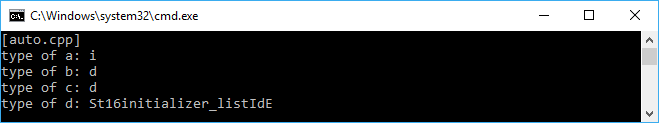As can be seen in the preceding snapshot, we are just given the first character of the data type, such as `i` for `integer`, `d` for `double`, and `St16initializer_listIdE` for `initializer_list<double>`, that is the last lowercase `d` character that stands for `double`.

### Note

We may have to enable the Run-Time Type Information (RTTI) feature in our compiler options to retrieve the data type object. However, GCC has enabled the feature by default. Also, the output of the use of the `typeid()` function depends on the compiler. We may get the raw type name or just a symbol as we did in the preceding example.

Besides, for variable, as we discussed earlier, the `auto` keyword can also be applied to a function to deduce a function's return type automatically. Suppose we have the following trivial function named `add()` to calculate the addition of two parameters:

```    int add(int i, int j)
{
return i + j;
}```

We can refactor the preceding method to use the `auto` keyword, as we can see in the following lines of code:

```    auto add(int i, int j)
{
return i + j;
}```

Similar to the auto-type variable, the compiler can decide the correct return type based on the returned value of the function. And, as shown in the preceding code, the function will indeed return the integer value since we just add two integer values.

Another feature that uses the `auto` keyword in modern C++ is trailing the return type syntax. By using this feature, we can specify the return type, the rest of the function prototype, or function signature. From the preceding code, we can refactor it to use the feature as follows:

```    auto add(int i, int j) -> int
{
return i + j;
}```

You might ask me why we have to specify the data type again after the arrow symbol (`->`), even though we have used the `auto` keyword. We will find the answer when we cover the `decltype` keyword in the next section. Also, by using this feature, we can now refactor the preceding `auto.cpp` code a little bit by modifying the syntax of the `main()` method, instead of the following syntax of `main()` function signature:

```    int main()
{
// The body of the function
}```

We can change the signature syntax into the following line of code:

```    auto main -> int
{
// The body of the function
}```

Now, we will see all of our code in this book using this trailing return type feature to apply the modern C++ syntax.

### Querying the type of an expression using the decltype keyword

We discussed in the preceding section that the `auto` keyword can automatically deduce the type of the variable based on the type of values it stores. The keyword can also deduce the function's return type based on the type of its return value. Now, let's combine the `auto` keyword and the `decltype` keyword to gain the power of modern C++.

Before we combine the two keywords, we will find out what the `decltype` keyword is used for--it is used for asking the type of an object or an expression. Let's take a look at the following several lines of trivial variable declaration:

```    const int func1();
const int& func2();
int i;

struct X { double d; };
const X* x = new X();```

Now, based on the preceding code, we can declare other variables using the `decltype` keyword as follows:

```    // Declaring const int variable
// using func1() type
decltype(func1()) f1;

// Declaring const int& variable
// using func2() type
decltype(func2()) f2;

// Declaring int variable
// using i type
decltype(i) i1;

// Declaring double variable
// using struct X type
decltype(x->d) d1; // type is double
decltype((x->d)) d2; // type is const double&```

As we can see in the preceding code, we can specify the type of an object based on another object's type. Now, let's suppose we need to refactor the preceding `add()` method to become a template. Without the `auto` and `decltype` keyword, we will have the following template implementation:

```    template<typename I, typename J, typename K>
{
return i + j;
}```

Fortunately, since the `auto` keyword can specify the return type of the function, which is a trailing return type, and the `decltype` keyword can deduce the type based on the expression, we can refactor the preceding template as follows:

```    template<typename I, typename J>
auto add(I i, J j) -> decltype(i + j)
{
return i + j;
}```

To prove, let's compile and run the following `decltype.cpp` code. We will use the following template to calculate the addition of two different value types--`integer` and `double`:

```    /* decltype.cpp */
#include <iostream>

// Creating template
template<typename I, typename J>
auto add(I i, J j) -> decltype(i + j)
{
return i + j;
}

auto main() -> int
{
std::cout << "[decltype.cpp]" << std::endl;

// Consuming the template
auto d = add<int, double>(2, 2.5);

// Displaying the preceding variables' type
std::cout << "result of 2 + 2.5: " << d << std::endl;

return 0;
}```

The compilation process should run smoothly without error. We will see the following output on the screen if we run the preceding code: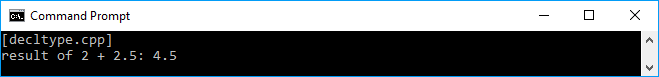As we can see, we have successfully combined the `auto` and `decltype` keyword to create a template simpler than we usually do before the modern C++ is announced.

### Pointing to a null pointer

Another new feature in modern C++ is a keyword named `nullptr` that replaces the `NULL` macro to represent a null pointer. Now, there's no ambiguity in the use of the `NULL` macro for zero numeric or a null pointer. Let's suppose we have the following two method's signature in our declaration:

```    void funct(const char *);
void funct(int)```

The former function will pass a pointer as the argument and the latter will pass the integer number as its argument. Then, we invoke the `funct()` method and pass the `NULL` macro as the parameter, as shown here:

`    funct(NULL);`

What we intend to call is the former function. However, since we pass the `NULL` parameters, which is basically defined as `0`, the latter function will be invoked. In modern C++, we can use the `nullptr` keyword to ensure that we will pass a null pointer to the argument. The invocation of the `funct()` method should be as follows:

`    funct(nullptr);`

Now the compiler will invoke the former function since it passes a null pointer to the argument, and this is what we expect. There will be no ambiguity anymore, and it will avoid unnecessary future problems.

### Returning an iterator using non-member begin() and end() function

Prior to modern C++, to iterate a sequence, we call the `begin()` and `end()` member method of each container. For array, we can iterate its element by iterating the index. Since C++11, the language has a non-member function--`begin()` and `end()`--to retrieve the iterator of the sequence. Let's suppose we have an array of the following elements:

`    int arr[] = { 0, 1, 2, 3, 4, 5, 6, 7, 8, 9 };`

When the language doesn't have the `begin()` and `end()` function, we need to iterate the elements of the array using the index we can see in the following lines of code:

```    for (unsigned int i = 0; i < sizeof(arr)/sizeof(arr); ++i)
// Do something to the array```

Fortunately, using the `begin()` and `end()` function, we can refactor the preceding `for` loop to become as follows:

```    for (auto i = std::begin(arr); i != std::end(arr); ++i)
// Do something to the array```

As we can see, the use of the `begin()` and `end()` function creates a compact code since we don't need to worry about the length of the array because the iterator pointer of `begin()` and `end()` will do it for us. For comparison, let's take a look at the following `begin_end.cpp` code:

```    /* begin_end.cpp */
#include <iostream>

auto main() -> int
{
std::cout << "[begin_end.cpp]" << std::endl;

// Declaring an array
int arr[] = { 0, 1, 2, 3, 4, 5, 6, 7, 8, 9 };

// Displaying the array elements
// using conventional for-loop
std::cout << "Displaying array element using conventional for-
loop";
std::cout << std::endl;
for (unsigned int i = 0; i < sizeof(arr)/sizeof(arr); ++i)
std::cout << arr[i] << " ";
std::cout << std::endl;

// Displaying the array elements
// using non-member begin() and end()
std::cout << "Displaying array element using non-member begin()
and end()";
std::cout << std::endl;
for (auto i = std::begin(arr); i != std::end(arr); ++i)
std::cout << *i << " ";
std::cout << std::endl;

return 0;
}```

To prove the preceding code, we can compile the code, and, when we run it, the following output should be displayed on the console screen: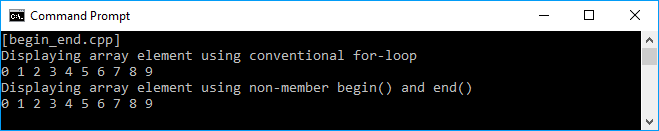As we can see in the screenshot, we've got the exact same output when we use the conventional `for-loop` or `begin()` and `end()` functions.

### Iterating over collections using range-based for loops

In the modern C++, there is a new feature that is augmented to support the `for-each` technique to iterate over collections. This feature is useful if you want to do something to the elements of a collection or array without caring about the number of elements or the indexes. The syntax of the feature is also simple. Suppose we have an array named `arr` and we want to iterate each element using the `range-based for loop` technique; we can use the following syntax:

```    for (auto a : arr)
// Do something with a```

So, we can refactor our preceding `begin_end.cpp` code to use `range-based for loop` as we can see in the following code:

```    /* range_based_for_loop.cpp */
#include <iostream>

auto main() -> int
{
std::cout << "[range_based_for_loop.cpp]" << std::endl;

// Declaring an array
int arr[] = {0, 1, 2, 3, 4, 5, 6, 7, 8, 9};

// Displaying the array elements
// using non-member begin() and end()
std::cout << "Displaying array element using range-based for
loop";
std::cout << std::endl;
for (auto a : arr) std::cout << a << " ";
std::cout << std::endl;

return 0;
}```

The syntax we see in the preceding code is simpler now. If we compile the preceding code, we should find no error and, if we run the code, we should see the following output on the console screen: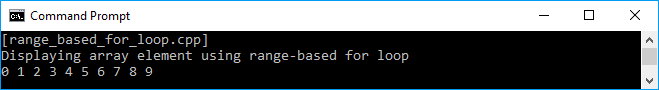We now have a new technique to iterate over the collection without caring about the indexes of the collection. We will keep using it in this book.

## Leveraging the use of C++ language with the C++ Standard Libraries

The C++ Standard Libraries are a powerful set of classes and functions that have many capabilities needed to create an application. They are controlled by the C++ ISO Standard Committee and is influenced by the Standard Template Libraries (STL), which were the generic libraries before C++11 was introduced. All features in Standard Libraries are declared in `std namespace` and no headers end in `.h` anymore (except 18 headers of the ISO C90 C Standard Library that is incorporated into the C++ Standard Libraries).

There are several header files containing the declaration of the C++ Standard Libraries. However, it is almost impossible to discuss all header files in these tiny chapters. We will, therefore, talk about some features that we will use most in our daily coding activities.

### Placing any objects in the container

Container is an object that is used to store other objects and manage the memory that is used by the objects it contains. An array is a new feature added in C++11 to store the collection of specific data types. It is a sequence container since it stores the same data type objects and arranges them linearly. Let's take a look at the following code snippet:

```    /* array.cpp */
#include <array>
#include <iostream>

auto main() -> int
{
std::cout << "[array.cpp]" << std::endl;

// Initializing an array containing five integer elements
std::array<int, 10> arr = { 0, 1, 2, 3, 4, 5, 6, 7, 8, 9 };

// Displaying the original elements of the array
std::cout << "Original Data : ";
for(auto a : arr) std::cout << a << " ";
std::cout << std::endl;

// Modifying the content of
// the 1st and 3rd element of the array
arr = 9;
arr = 7;

// Displaying the altered array elements
std::cout << "Manipulated Data: ";
for(auto a : arr) std::cout << a << " ";
std::cout << std::endl;

return 0;
}```

As we can see in the preceding code, we instance a new array named `arr`, set its length as `10`, and only approve the `int` element. As we can guess, the output of the code is a line of numbers `0` through `9`, which is shown in the original data, and the other line will show the altered data, as we can see in the following screenshot: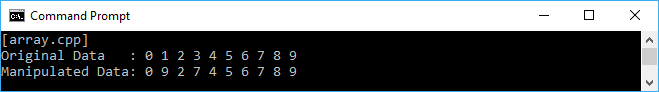### Note

There is no performance issue if we declare an array using `std::array`; we use in the `array.cpp` code and compare it with a usual array as we use in the `begin_end.cpp` code. However, in modern C++, we are given a new array declaration that has a friendly value semantic, so that it can be passed to or returned from functions by value. Also, the interface of this new array declaration makes it more convenient to find the size, and use it with Standard Template Library (STL)-style iterator-based algorithms.

It is good to use an array as the container since we can store the data and manipulate them. We can also sort and find a specific element if we want. However, since the array is a compile-time non-resizable object, we have to decide the size of the array we intend to use at the very beginning as we cannot change the size later. In other words, we cannot insert or remove the element from the existing array. As a solution to this problem, and for the best practice of using the container as well, we can now use a `vector` to store our collection. Let's take a look at the following code:

```    /* vector.cpp */
#include <vector>
#include <iostream>

auto main() -> int
{
std::cout << "[vector.cpp]" << std::endl;

// Initializing a vector containing three integer elements
std::vector<int> vect = { 0, 1, 2 };

// Displaying the original elements of the vector
std::cout << "Original Data : ";
for (auto v : vect) std::cout << v << " ";
std::cout << std::endl;

vect.push_back(3);
vect.push_back(4);

// Displaying the elements of the new vector
// and reverse the order
std::cout << "New Data Added : ";
for (auto v : vect) std::cout << v << " ";
std::cout << std::endl;

// Modifying the content of
// the 2nd and 4th element of the vector
vect.at(2) = 5;
vect.at(4) = 6;

// Displaying the altered array elements
std::cout << "Manipulate Data: ";
for (auto v : vect) std::cout << v << " ";
std::cout << std::endl;

return 0;
}```

Now, we have a `vector` instance in our preceding code instead of an `array` instance. As we can see, we give an additional value for the `vector` instance using the `push_back()` method. We can add the value anytime we want. The manipulation of each element is also easier since `vector` has an `at()` method that returns a reference to the element of the specific index. The following screenshot is what we will see as the output when running the code: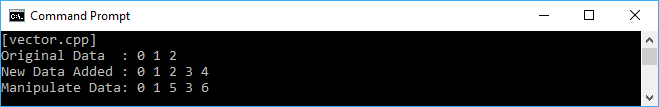### Note

It is better to always use the `at()` method instead of the `[]` operator when we want to access the specific element by its index in a `vector` instance. It's because, when we accidentally access the out of range position, the `at()` method will throw an `out_of_range` exception. Otherwise, the `[]` operator will give undefined behavior.

### Using algorithms

We can sort the elements of the collection we have in `array` or `vector`, as well as find specific content of the element. For these purposes, we have to use the algorithm feature provided by the C++ Standard Library. Let's take a look at the following code to demonstrate the sorting element capability in the algorithm feature:

```    /* sort.cpp */
#include <vector>
#include <algorithm>
#include <iostream>

bool comparer(int a, int b)
{
return (a > b);
}

auto main() -> int
{
std::cout << "[sort.cpp]" << std::endl;

// Initializing a vector containing several integer elements
std::vector<int> vect = { 20, 43, 11, 78, 5, 96 };

// Displaying the original elements of the vector
std::cout << "Original Data : ";
for (auto v : vect)
std::cout << v << " ";
std::cout << std::endl;

// Sorting the vector element ascending
std::sort(std::begin(vect), std::end(vect));

// Displaying the ascending sorted elements
// of the vector
std::cout << "Ascending Sorted : ";
for (auto v : vect)
std::cout << v << " ";
std::cout << std::endl;

// Sorting the vector element descending
// using comparer
std::sort(std::begin(vect), std::end(vect), comparer);

// Displaying the descending sorted elements
// of the vector
std::cout << "Descending Sorted: ";
for (auto v : vect)
std::cout << v << " ";
std::cout << std::endl;

return 0;
}```

As we see in the preceding code, we invoked the `sort()` method twice. First, we just supplied the range of the elements we wanted to sort. Then we added the comparison function, `comparer()`, to be provided to the `sort()` method to gain more flexibility the method has. The output we will see on the console from the preceding code is as follows: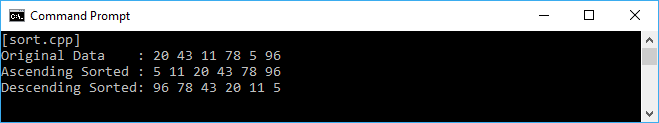From the preceding screenshot, we can see that we have six elements in a `vector` at the beginning. We then sort the elements of the vector using a simple `sort()` method. Then, we invoke the `sort()` method again, but instead of a simple `sort()` method, we now supply `comparer()` to the `sort()` method. As a result, the vector elements will be sorted descendingly since the `comparer()` function looks for the greater value from two inputs.

Now, let's move to another capability the algorithm feature has, which is finding a particular element. Let's suppose we have the `Vehicle` class in our code. It has two private fields named `m_vehicleType` and `m_totalOfWheel`, and we can retrieve the value from the getter methods named `GetType()` and `GetNumOfWheel()` respectively. It also has two constructors, which are the default constructor and the user-defined one. The declaration of the class should be as follows:

```    /* vehicle.h */
#ifndef __VEHICLE_H__
#define __VEHICLE_H__

#include <string>

class Vehicle
{
private:
std::string vehicleType;
int totalOfWheel;

public:
Vehicle(
const std::string &type,
int _wheel);
Vehicle();
~Vehicle();
std::string GetType() const {return vehicleType;}
};

#endif // End of __VEHICLE_H__```

The implementation of the `Vehicle` class is as follows:

```    /* vehicle.cpp */
#include "vehicle.h"

using namespace std;

// Constructor with default value for
// m_vehicleType and m_totalOfWheel
Vehicle::Vehicle() : m_totalOfWheel(0)
{
}

// Constructor with user-defined value for
// m_vehicleType and m_totalOfWheel
Vehicle::Vehicle( const string &type, int wheel) :
m_vehicleType(type),
m_totalOfWheel(wheel)
{
}

// Destructor
Vehicle::~Vehicle()
{
}```

We will store a collection of `Vehicle` in the `vector` container, and then we will search for some elements based on its property. The code will be as follows:

```    /* find.cpp */
#include <vector>
#include <algorithm>
#include <iostream>
#include "../vehicle/vehicle.h"

using namespace std;

bool TwoWheeled(const Vehicle &vehicle)
{
return _vehicle.GetNumOfWheel() == 2 ?
true : false;
}

auto main() -> int
{
cout << "[find.cpp]" << endl;

// Initializing several Vehicle instances
Vehicle car("car", 4);
Vehicle motorcycle("motorcycle", 2);
Vehicle bicycle("bicycle", 2);
Vehicle bus("bus", 6);

// Assigning the preceding Vehicle instances to a vector
vector<Vehicle> vehicles = { car, motorcycle, bicycle, bus };

// Displaying the elements of the vector
cout << "All vehicles:" << endl;;
for (auto v : vehicles)
std::cout << v.GetType() << endl;
cout << endl;

// Displaying the elements of the vector
// which are the two-wheeled vehicles
cout << "Two-wheeled vehicle(s):" << endl;;
auto tw = find_if(
begin(vehicles),
end(vehicles),
TwoWheeled);
while (tw != end(vehicles))
{
cout << tw->GetType() << endl ;
tw = find_if(++tw, end(vehicles), TwoWheeled);
}
cout << endl;

// Displaying the elements of the vector
// which are not the two-wheeled vehicles
cout << "Not the two-wheeled vehicle(s):" << endl;;
auto ntw = find_if_not(begin(vehicles),
end(vehicles),
TwoWheeled);
while (ntw != end(vehicles))
{
cout << ntw->GetType() << endl ;
ntw = find_if_not(++ntw, end(vehicles), TwoWheeled);
}

return 0;
}```

As we can see, we instance four `Vehicle` objects, then store them in `vector`. There, we try to find the vehicle that has two wheels. The `find_if()` function is used for this purpose. We also have the `TwoWheeled()` method to provide the comparison value. Since we are finding the two-wheeled vehicle, we will inspect the `totalOfWheel` variable in the `Vehicle` class by invoking the `GetNumOfWheel()` method. In contrast, if we want to find the element that doesn't conform to the comparison value, we can use the `find_if_not()` function, which had been added in C++11. The output we get should look like this: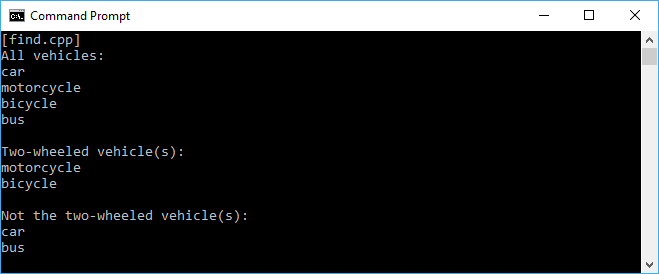### Note

As we can see in the `vehicle.cpp` code and `find.cpp` code, we now add the `using namespace std;` line in the `*.cpp` files. We do this to make our coding activity become more productive since we don't have to type many words. In contrast, in `vehicle.h`, we still using `std::` followed by the methods or properties name rather than use the std namespace at the beginning. It's best practice to not declare `using namespace` in header files since the header files are the files we will deliver if we create some libraries for instances. The user of our library may have another method with the same name as the function our library has. It will definitely create conflict between these two functions.

Another algorithm feature we will use most is the `for_each` loop. Instead of using the `for` loop, the use of the `for_each` loop will make our code more concise in many cases. It's also simpler and less error prone than a `for` loop because we can define a specific function for the `for_each` loop. Now let's refactor our previous code to use the `for_each` loop. The code is written as follows:

```    /* for_each.cpp */
#include <vector>
#include <algorithm>
#include <iostream>
#include "vehicle.h"

using namespace std;

void PrintOut(const Vehicle &vehicle)
{
cout << vehicle.GetType() << endl;
}

auto main() -> int
{
cout << "[for_each.cpp]" << endl;

// Initializing several Vehicle instances
Vehicle car("car", 4);
Vehicle motorcycle("motorcycle", 2);
Vehicle bicycle("bicycle", 2);
Vehicle bus("bus", 6);

// Assigning the preceding Vehicle instances to a vector
vector<Vehicle> vehicles = { car, motorcycle, bicycle, bus };

// Displaying the elements of the vector
cout << "All vehicles:" << endl;
for_each(begin(vehicles), end(vehicles), PrintOut);

return 0;
}```

Now, with the `for_each` loop, we have a clearer code. We only need to provide the first and last iterator and then pass a function--the `PrintOut()` function in this case--that will be invoked in each element in the range.

## Simplifying the function notation using a Lambda expression

The Lambda expression is an anonymous notation that represents something that performs an operation or calculation. In functional programming, the Lambda expression is useful to produce the first class and pure function, which we will discuss in separate chapters in this book. For now, let's familiarize ourselves with this new feature introduced in C++11 by investigating three basic parts of the Lambda expression:

• capturing list: []
• parameter list: ()
• body: {}

The order of these three basic parts is as follows:

`    [](){} `

The capturing list part is also used as a mark to identify the Lambda expression. It is a placeholder to value to be involved in the expression. The only capture defaults are the ampersand symbol (`&`), which will implicitly capture the automatic variables by reference, and the equal sign (`=`), which will implicitly capture the automatic variables by copy (we will discuss it further in the upcoming section). The parameter list is similar to the capturing list in every function where we can pass the value to it. The body is the implementation of the function itself.

### Using the Lambda expression for a tiny function

Imagine we have a tiny one-line function that we invoke only once. It's better if we write the operation of that function directly when we need it. We actually had this function in our previous example when discussing the C++ Standard Library. Just go back to the `for_each.cpp` file and we will find the `PrintOut()` function that is only invoked once by `for_each()`. We can make this `for_each` loop more readable if we use Lambda. Let's take a look at the following code snippet to examine how we refactor the `for_each.cpp` file:

```    /* lambda_tiny_func.cpp */
#include <vector>
#include <algorithm>
#include <iostream>
#include "../vehicle/vehicle.h"

using namespace std;

auto main() -> int
{
cout << "[lambda_tiny_func.cpp]" << endl;

// Initializing several Vehicle instances
Vehicle car("car", 4);
Vehicle motorcycle("motorcycle", 2);
Vehicle bicycle("bicycle", 2);
Vehicle bus("bus", 6);

// Assigning the preceding Vehicle instances to a vector
vector<Vehicle> vehicles = { car, motorcycle, bicycle, bus };

// Displaying the elements of the vector
// using Lambda expression
cout << "All vehicles:" << endl;
for_each(
begin(vehicles),
end(vehicles),
[](const Vehicle &vehicle){
cout << vehicle.GetType() << endl;
});

return 0;
}```

As we can see, we have transformed the `PrintOut()` function that we used in the `for_each.cpp` file into a Lambda expression and passed it to the `for_each` loop. It will indeed give the same output as the `for_each.cpp` file does. However, now our code becomes more concise and readable.

### Using the Lambda expression for multiline functions

The Lambda expression can also be used for multiline functions, so we can put the body of the function on it. This will make our code more readable as well. Let's make a new code. In that code, we will have an integer collection and an intent to inspect whether the selected element is the prime number or not. We can make a separate function, for instance, `PrintPrime()`, then invoke it. However, since the prime number checking operation is called only once, it's more readable if we transform it into a Lambda expression. The code should look like this:

```    /* lambda_multiline_func.cpp */
#include <vector>
#include <algorithm>
#include <iostream>

using namespace std;

auto main() -> int
{
cout << "[lambda_multiline_func.cpp]" << endl;

// Initializing a vector containing integer element
vector<int> vect;
for (int i = 0; i < 10; ++i)
vect.push_back(i);

// Displaying whether or not the element is prime number
for_each(
begin(vect),
end(vect),
[](int n) {
cout << n << " is";
if(n < 2)
{
if(n == 0)
cout << " not";
}
else
{
for (int j = 2; j < n; ++j)
{
if (n % j == 0)
{
cout << " not";
break;
}
}
}

cout << " prime number" << endl;
});

return 0;
}```

The output we should see on the screen is as follows: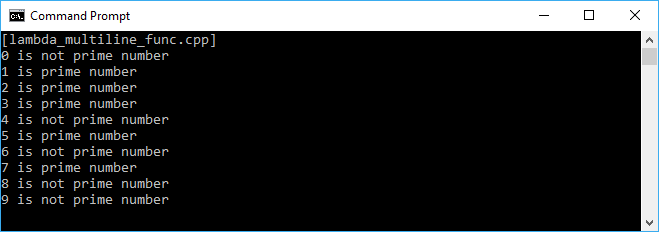As we can see in the preceding screenshot, we have successfully identified the prime number by using the Lambda expression.

### Returning a value from the Lambda expression

Our two preceding samples of the Lambda expression are just for the purpose to print on console. It means the function does not need to return any value. However, we can ask the Lambda expression to return a value for an instance if we do the calculation inside the function and return the calculation result. Let's take a look at the following code to examine the use of this Lambda:

```    /* lambda_returning_value.cpp */
#include <vector>
#include <algorithm>
#include <iostream>

using namespace std;

auto main() -> int
{
cout << "[lambda_returning_value.cpp]" << endl;

// Initializing a vector containing integer element
vector<int> vect;
for (int i = 0; i < 10; ++i)
vect.push_back(i);

// Displaying the elements of vect
cout << "Original Data:" << endl;
for_each(
begin(vect),
end(vect),
[](int n){
cout << n << " ";
});
cout << endl;

// Creating another vect2 vector
vector<int> vect2;
// Resize the size of vect2 exactly same with vect
vect2.resize(vect.size());
// Doubling the elements of vect and store to vect2
transform(
begin(vect),
end(vect),
begin(vect2),
[](int n) {
return n * n;
});

// Displaying the elements of vect2
cout << "Squared Data:" << endl;
for_each(
begin(vect2),
end(vect2),
[](int n) {
cout << n << " ";
});
cout << endl;

// Creating another vect3 vector
vector<double> vect3;
// Resize the size of vect3 exactly same with vect
vect3.resize(vect.size());
// Finding the average of the elements of vect
// and store to vect2
transform(
begin(vect2),
end(vect2),
begin(vect3),
[](int n) -> double {
return n / 2.0;
});

// Displaying the elements of vect3
cout << "Average Data:" << endl;
for_each(
begin(vect3),
end(vect3),
[](double d) {
cout << d << " ";
});
cout << endl;

return 0;
}```

When we use the `transform()` method in the preceding code, we have a Lambda expression that returns a value from the calculation of `n * n`. However, there's no return type stated in the expression. This is because we can omit the statement of the return type since the compiler has understood that the expression will return an `integer` value. So, after we have another vector, `vect2`, which has the same size as `vect`, we can invoke the `transform()` method along with the Lambda expression, and the value of `vect` will be doubled and stored in `vect2`.

We can, if we want to, specify the return type to the Lambda expression. As we can see in the preceding code, we transformed the `vect3` vector based on all values of the `vect` vector, but now we specify the return type to `double` using the arrow symbol (`->`). The result of the preceding code should be like the following screenshot:As we can see from the preceding screenshot, we have successfully found the doubled and average result using the Lambda expression.

### Capturing a value to the Lambda expression

In our previous Lambda expression examples, we keep the capturing part and the square bracket (`[]`) empty since the Lambda doesn't capture anything and doesn't have any extra member variable in the anonymous object generated by the compiler. We can also specify the object we want to capture in the Lambda expression by specifying it in this square bracket. Let's take a look at the following piece of code to go through the discussion:

```    /* lambda_capturing_by_value.cpp */
#include <vector>
#include <algorithm>
#include <iostream>

using namespace std;

auto main() -> int
{
cout << "[lambda_capturing_by_value.cpp]" << endl;

// Initializing a vector containing integer element
vector<int> vect;
for (int i = 0; i < 10; ++i)
vect.push_back(i);

// Displaying the elements of vect
cout << "Original Data:" << endl;
for_each(
begin(vect),
end(vect),
[](int n){
cout << n << " ";
});
cout << endl;

// Initializing two variables
int a = 2;
int b = 8;

// Capturing value explicitly from the two variables
cout << "Printing elements between " << a;
cout << " and " << b << " explicitly [a,b]:" << endl;
for_each(
begin(vect),
end(vect),
[a,b](int n){
if (n >= a && n <= b)
cout << n << " ";
});
cout << endl;

// Modifying variable a and b
a = 3;
b = 7;

// Capturing value implicitly from the two variables
cout << "printing elements between " << a;
cout << " and " << b << " implicitly[=]:" << endl;
for_each(
begin(vect),
end(vect),
[=](int n){
if (n >= a && n <= b)
cout << n << " ";
});
cout << endl;

return 0;
}```

In the preceding code, we will try to capture the value in the Lambda expression, explicitly and implicitly. Let's suppose we have two variables, `a` and `b`, and we want to explicitly capture the values, we can specify them in the Lambda expression using the `[a,b]` statement, and then using the values inside the function body. Moreover, if we wish to capture the value implicitly, just use `[=]` for the capturing part and then the expression will know which variable we intend to use when we specify them in the function body. If we run the preceding code, we will get the following output on the screen: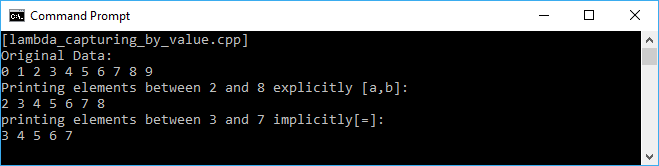We can also mutate the state of the values we capture without modifying the value outside the Lambda expression function body. For this purpose, we can use the same techniques as used previously, and add the `mutable` keyword as shown in the following block of code:

```    /* lambda_capturing_by_value_mutable.cpp */
#include <vector>
#include <algorithm>
#include <iostream>

using namespace std;

auto main() -> int
{
cout << "[lambda_capturing_by_value_mutable.cpp]" << endl;

// Initializing a vector containing integer element
vector<int> vect;
for (int i = 0; i < 10; ++i)
vect.push_back(i);

// Displaying the elements of vect
cout << "Original Data:" << endl;
for_each(
begin(vect),
end(vect),
[](int n){
cout << n << " ";
});
cout << endl;

// Initializing two variables
int a = 1;
int b = 1;

// Capturing value from the two variables
// without mutate them
for_each(
begin(vect),
end(vect),
[=](int& x) mutable {
const int old = x;
x *= 2;
a = b;
b = old;
});

// Displaying the elements of vect
cout << "Squared Data:" << endl;
for_each(
begin(vect),
end(vect),
[](int n) {
cout << n << " ";
});
cout << endl << endl;

// Displaying value of variable a and b
cout << "a = " << a << endl;
cout << "b = " << b << endl;

return 0;
}```

The preceding code will double the element of the `vect` vector. It uses capturing by value in the Lambda expression and also the `mutable` keyword. As we can see, we passed the vector element by reference `(int& x)` and multiplied it by two, then changed the value of `a` and `b`. However, since we use the `mutable` keyword, the final result of `a` and `b` will remain the same, although, we have passed the vector by reference. The output on the console looks like the following screenshot: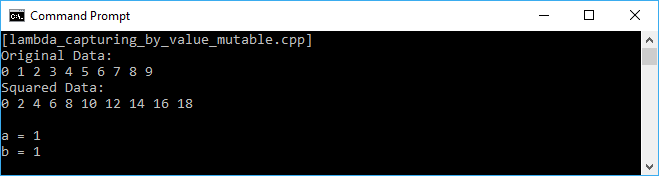If we want to change the value of the `a` and `b` variables, we have to use the Lambda expression to capture by reference. We can do this by passing the reference to the angle bracket in the Lambda expression, for instance, `[&a, &b]`. For more detail, let's take a look at the following piece of code:

```    /* lambda_capturing_by_reference.cpp */
#include <vector>
#include <algorithm>
#include <iostream>

using namespace std;

auto main() -> int
{
cout << "[lambda_capturing_by_reference.cpp]" << endl;

// Initializing a vector containing integer element
vector<int> vect;
for (int i = 0; i < 10; ++i)
vect.push_back(i);

// Displaying the elements of vect
cout << "Original Data:" << endl;
for_each(
begin(vect),
end(vect),
[](int n){
cout << n << " ";
});
cout << endl;

// Initializing two variables
int a = 1;
int b = 1;

// Capturing value from the two variables
// and mutate them
for_each(
begin(vect),
end(vect),
[&a, &b](int& x){
const int old = x;
x *= 2;
a = b;
b = old;
});

// Displaying the elements of vect
cout << "Squared Data:" << endl;
for_each(
begin(vect),
end(vect),
[](int n) {
cout << n << " ";
});
cout << endl << endl;

// Displaying value of variable a and b
cout << "a = " << a << endl;
cout << "b = " << b << endl;

return 0;
}```

The preceding code has the same behavior with the `lambda_capturing_by_value_mutable.cpp` file that will double the element of the `vect` vector. However, by capturing by reference, it now also modifies the value of `a` and `b` when they are processed in the `for_each` loop. The `a` and `b` values will be changed at the end of the code, as we can see in the following screenshot: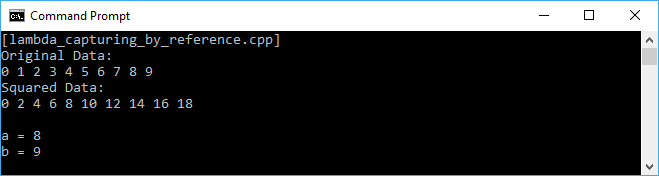### Preparing the value using initialization captures

Another great feature of the Lambda expression coming up in C++14 is its initialization captures. The expression can capture a value of the variable and assign it to the expression's variable. Let's take a look at the following piece of code implementing the initialization captures:

```    /* lambda_initialization_captures.cpp */
#include <iostream>

using namespace std;

auto main() -> int
{
cout << "[lambda_initialization_captures.cpp]" << endl;

// Initializing a variable
int a = 5;
cout << "Initial a = " << a << endl;

// Initializing value to lambda using the variable
auto myLambda = [&x = a]() { x += 2; };

// Executing the Lambda
myLambda();

// Displaying a new value of the variable
cout << "New a = " << a << endl;

return 0;
}```

As we can see in the preceding code, we have an int variable named `a` with the value `5`. The Lambda expression, `myLambda`, then captures the `a` value and executes it in the code. The result is that now the `a` value will be `7` since it is added by `2`. The following output screenshot should appear in our console window when we run the preceding code: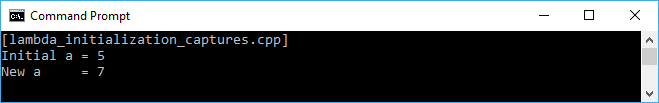From the preceding snapshot, we see that we can prepare the value to be included in the calculation inside the Lambda expression.

### Writing a generic Lambda expression to be used many times with many different data types

Before C++14, we have to specifically state the type of the parameter list. Fortunately, now in C++14, Lambda expressions accept `auto` as a valid parameter type. Therefore, we can now build a generic Lambda expression as demonstrated in the following code. In that code, we have only one Lambda expression to find out which is the greatest value between two numbers passed to the expression. We will use the `auto` keyword in parameter declaration so it can be passed by any data type. Therefore, the `findMax()` function parameters can be passed by both the `int` and `float` data type. The code should be as follows:

```    /* lambda_expression_generic.cpp */
#include <iostream>

using namespace std;

auto main() -> int
{
cout << "[lambda_expression_generic.cpp]" << endl;

// Creating a generic lambda expression
auto findMax = [](auto &x, auto &y){
return x > y ? x : y; };

// Initializing various variables
int i1 = 5, i2 = 3;
float f1 = 2.5f, f2 = 2.05f;

// Consuming generic lambda expression
// using integer data type
cout << "i1 = 5, i2 = 3" << endl;
cout << "Max: " << findMax(i1, i2) << endl << endl;

// Consuming generic lambda expression
// using double data type
cout << "f1 = 2.5f, f2 = 2.05f" << endl;
cout << "Max: " << findMax(f1, f2) << endl << endl;

return 0;
}```

The output we will see on the console should be as follows: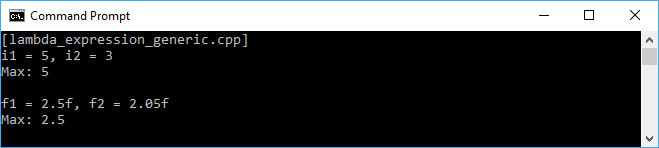### Note

The C++17 language plans to introduce two new features for the Lambda expression--they are capturing `*this`, which allows the expression to capture the enclosing object by copy, and the `constexpr` Lambda expressions, which allows us to use the result of the Lambda expressions and generate `constexpr` objects at compile time. However, since C++17 has not been released yet, we cannot try it for now.

## Avoiding manual memory management with smart pointers

The smart pointers are highly useful and have an essential knowledge in using C++ efficiently. C++11 added many new abilities for the smart pointer we can find in the `memory` header file. For a long time, before C++11, we used `auto_ptr` as a smart pointer. However, it was quite unsafe since it had incompatible copy semantics. It's also deprecated now, and we should not use it anymore. Fortunately, C++ has presented `unique_ptr`, which has a similar functionality, but with additional features, such as adding `deleters` and support for arrays. Anything we can do with `auto_pt`, we can and should do with `unique_ptr` instead. We will discuss `unique_ptr` in depth along with other new smart pointers in C++11--`shared_ptr` and `weak_ptr`.

### Replacing a raw pointer using unique_ptr

The next pointer we will see is the `unique_ptr` pointer. It is fast, efficient, and a near drop-in replacement for raw or naked pointers. It provides exclusive ownership semantics, which exclusively owns the object that it points to. By its exclusiveness, it can destroy the object when its destructor is called if it has a non-null pointer. It also cannot be copied due to its exclusiveness. It has no copy constructor and copy assignment. Although it cannot be copied, it can be moved since it provides a move constructor and a move assignment.

These are the methods we can use to construct `unique_ptr`:

```    auto up1 = unique_ptr<int>{};
auto up2 = unique_ptr<int>{ nullptr };
auto up3 = unique_ptr<int>{ new int { 1234 } };```

Based on the preceding code, `up1` and `up2` will construct two new `unique_ptr` that point to nothing (null), whereas `up3` will point to the address that holds the `1234` value. However, C++14 adds a new library function to construct `unique_ptr`, that is, `make_unique`. So, we can construct a new `unique_ptr` pointer as follows:

`    auto up4 = make_unique<int>(1234);`

The `up4` variable will also point to the address that holds the `1234` value.

Now, let's take a look at the following block of code:

```    /* unique_ptr_1.cpp */
#include <memory>
#include <iostream>

using namespace std;

struct BodyMass
{
int Id;
float Weight;

BodyMass(int id, float weight) :
Id(id),
Weight(weight)
{
cout << "BodyMass is constructed!" << endl;
cout << "Id = " << Id << endl;
cout << "Weight = " << Weight << endl;
}

~BodyMass()
{
cout << "BodyMass is destructed!" << endl;
}
};

auto main() -> int
{
cout << "[unique_ptr_1.cpp]" << endl;
auto myWeight = make_unique<BodyMass>(1, 165.3f);
cout << endl << "Doing something!!!" << endl << endl;
return 0;
}```

We try to construct a new `unique_ptr` pointer that points to the address that holds a `BodyMass` data type. In `BodyMass`, we have a constructor as well as a destructor. Now, let's see how the `unique_ptr` pointer works by running the preceding code. The output we get on the screen should be like the following screenshot: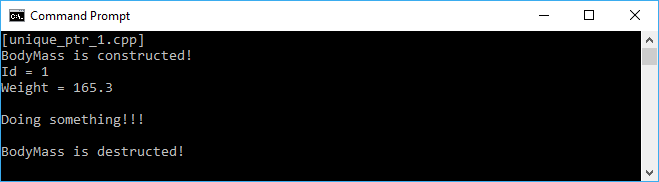As we can see in the preceding screenshot, the constructor is invoked when we construct `unique_ptr`. Moreover, unlike the traditional C++ language, where we have to free the memory up when we use a pointer, in modern C++, the memory will be freed up automatically when it is out of scope. We can see that the destructor of `BodyMass` is invoked when the program exits, which means `myWeight` is out of scope.

Now, let's test the exclusiveness of `unique_ptr` by analyzing the following code snippet:

```    /* unique_ptr_2.cpp */
#include <memory>
#include <iostream>

using namespace std;

struct BodyMass
{
int Id;
float Weight;

BodyMass(int id, float weight) :
Id(id),
Weight(weight)
{
cout << "BodyMass is constructed!" << endl;
cout << "Id = " << Id << endl;
cout << "Weight = " << Weight << endl;
}

BodyMass(const BodyMass &other) :
Id(other.Id),
Weight(other.Weight)
{
cout << "BodyMass is copy constructed!" << endl;
cout << "Id = " << Id << endl;
cout << "Weight = " << Weight << endl;
}

~BodyMass()
{
cout << "BodyMass is destructed!" << endl;
}
};

auto main() -> int
{
cout << "[unique_ptr_2.cpp]" << endl;

auto myWeight = make_unique<BodyMass>(1, 165.3f);

// The compiler will forbid to create another pointer
// that points to the same allocated memory/object
// since it's unique pointer
//auto myWeight2 = myWeight;

// However, we can do the following expression
// since it actually copies the object that has been allocated
// (not the unique_pointer)
auto copyWeight = *myWeight;

return 0;
}```

As we can see in the preceding code, we see that we can't assign the `unique_ptr` instance to another pointer since it will break the exclusiveness of `unique_ptr`. The compiler will throw an error if we make the following expression:

`    auto myWeight2 = myWeight;`

However, we can assign the value of the `unique_ptr` to another object since it has been allocated. To prove it, we have added a copy constructor to log when the following expression is executed:

`    auto copyWeight = *myWeight;`

If we run the preceding `unique_ptr_2.cpp` code, we will see the following output on the screen: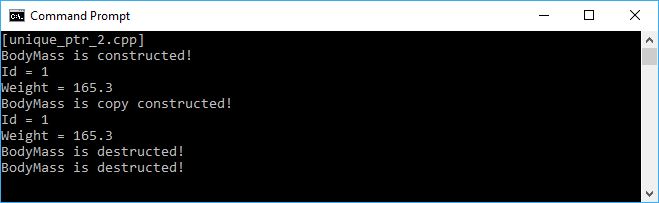As we can see in the preceding screenshot, the copy constructor is called when the copy assignment is executed. It proves that we can copy the value of the `unique_ptr` object but not the object itself.

As we discussed earlier, `unique_ptr` has moved the constructor, although it has no copy constructor. The use of this construction can be found in the following piece of code:

```    /* unique_ptr_3.cpp */
#include <memory>
#include <iostream>

using namespace std;

struct BodyMass
{
int Id;
float Weight;

BodyMass(int id, float weight) :
Id(id),
Weight(weight)
{
cout << "BodyMass is constructed!" << endl;
cout << "Id = " << Id << endl;
cout << "Weight = " << Weight << endl;
}

~BodyMass()
{
cout << "BodyMass is destructed!" << endl;
}
};

unique_ptr<BodyMass> GetBodyMass()
{
return make_unique<BodyMass>(1, 165.3f);
}

unique_ptr<BodyMass> UpdateBodyMass(
unique_ptr<BodyMass> bodyMass)
{
bodyMass->Weight += 1.0f;
return bodyMass;
}

auto main() -> int
{
cout << "[unique_ptr_3.cpp]" << endl;

auto myWeight = GetBodyMass();

cout << "Current weight = " << myWeight->Weight << endl;

myWeight = UpdateBodyMass(move(myWeight));

cout << "Updated weight = " << myWeight->Weight << endl;

return 0;
}```

In the preceding code, we have two new functions--`GetBodyMass()` and `UpdateBodyMass()`. We construct a new `unique_ptr` object from the `GetBodyMass()` function, then we update the value of its Weight using the `UpdateBodyMass()` function. We can see that we use the `move` function when we pass an argument to the `UpdateBodyMass()` function. It's because `unique_ptr` has no copy constructor, and it has to be moved in order to update the value of its property. The screen output of the preceding code is as follows: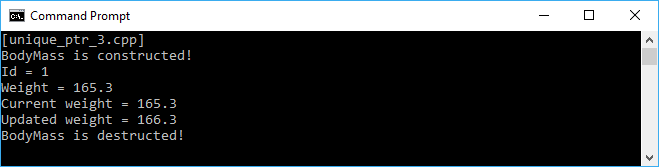### Sharing objects using shared_ptr

In contrast to `unique_ptr`, `shared_ptr` implements shared ownership semantics, so it offers the ability of copy constructor and copy assignment. Although they have a difference in the implementation, `shared_ptr` is actually the counted version of `unique_ptr`. We can call the `use_count()` method to find out the counter value of the `shared_ptr` reference. Each instance of the `shared_ptr` valid object is counted as one. We can copy the `shared_ptr` instance to other `shared_ptr` variables and the reference count will be incremented. When a `shared_ptr` object is destroyed, the destructor decrements the reference count. The object will be deleted only if the count reaches zero. Now let's examine the following `shared_ptr` code:

```    /* shared_ptr_1.cpp */
#include <memory>
#include <iostream>

using namespace std;

auto main() -> int
{
cout << "[shared_ptr_1.cpp]" << endl;

auto sp1 = shared_ptr<int>{};

if(sp1)
cout << "sp1 is initialized" << endl;
else
cout << "sp1 is not initialized" << endl;
cout << "sp1 pointing counter = " << sp1.use_count() << endl;
if(sp1.unique())
cout << "sp1 is unique" << endl;
else
cout << "sp1 is not unique" << endl;
cout << endl;

sp1 = make_shared<int>(1234);

if(sp1)
cout << "sp1 is initialized" << endl;
else
cout << "sp1 is not initialized" << endl;
cout << "sp1 pointing counter = " << sp1.use_count() << endl;
if(sp1.unique())
cout << "sp1 is unique" << endl;
else
cout << "sp1 is not unique" << endl;
cout << endl;

auto sp2 = sp1;

cout << "sp1 pointing counter = " << sp1.use_count() << endl;
if(sp1.unique())
cout << "sp1 is unique" << endl;
else
cout << "sp1 is not unique" << endl;
cout << endl;

cout << "sp2 pointing counter = " << sp2.use_count() << endl;
if(sp2.unique())
cout << "sp2 is unique" << endl;
else
cout << "sp2 is not unique" << endl;
cout << endl;

sp2.reset();

cout << "sp1 pointing counter = " << sp1.use_count() << endl;
if(sp1.unique())
cout << "sp1 is unique" << endl;
else
cout << "sp1 is not unique" << endl;
cout << endl;

return 0;
}```

Before we examine each line of the preceding code, let's take a look at the following output that should appear on the console window: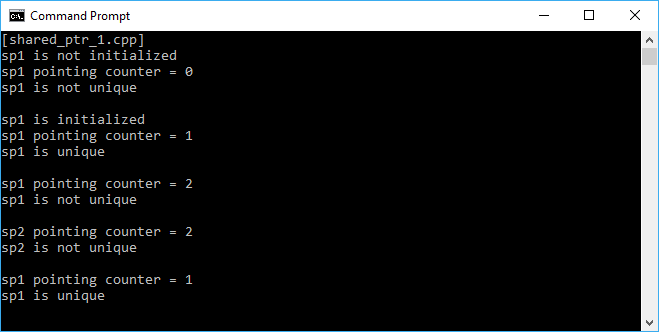First, we create a `shared_ptr` object named `sp1` without instantiating it. From the console, we see that `sp1` is not initialized and the counter is still `0`. It is also not unique since the pointer is pointed to nothing. We then construct `sp1` using the `make_shared` method. Now, `sp1` is initialized and the counter becomes `1`. It also becomes unique since it's only one of the `shared_ptr` object (proven by the value of the counter that is `1`). Next, we create another variable named `sp2`, and copy `sp1` to it. As a result, `sp1` and `sp2` now share the same object proven by the counter and the uniqueness value. Then, invoking the `reset()` method in `sp2` will destroy the object of `sp2`. Now, the counter of `sp1` becomes `1`, and it is unique again.

### Note

In the `shared_ptr_1.cpp` code, we declare the `unique_ptr` object using `shared_ptr<int>`, then invoke `make_shared<int>` to instance the pointer. It's because we just need to analyze the `shared_ptr` behavior. However, we should use `make_shared<>` for shared pointers since it has to keep the reference counter somewhere in memory and allocates the counter and memory for objects together instead of two separate allocations.

### Tracking the objects using a weak_ptr pointer

We have discussed the `shared_ptr` in the preceding section. The pointer is actually a little bit fat pointer. It logically points to two objects, the object being managed and the pointing counter using the `use_count()` method. Every `shared_ptr` basically has a strong reference count that prevents the object from being deleted and a weak reference count that does not prevent the object being deleted if the `shared_ptr` object's use count reaches 0, although we don't even use the weak reference count. For this reason, we can use only one reference count so we can use the `weak_ptr` pointer. The `weak_ptr` pointer refers to an object that is managed by `shared_ptr`. The advantage of `weak_ptr` is that it can be used to refer to an object, but we can only access it if the object still exists and without preventing the object from being deleted by some other reference holder if the strong reference count reaches zero. It is useful when we deal with data structures. Let's take a look at the following block of code to analyze the use of `weak_ptr`:

```    /* weak_ptr_1.cpp */
#include <memory>
#include <iostream>

using namespace std;

auto main() -> int
{
cout << "[weak_ptr_1.cpp]" << endl;

auto sp = make_shared<int>(1234);

auto wp = weak_ptr<int>{ sp };

if(wp.expired())
cout << "wp is expired" << endl;
else
cout << "wp is not expired" << endl;
cout << "wp pointing counter = " << wp.use_count() << endl;
if(auto locked = wp.lock())
cout << "wp is locked. Value = " << *locked << endl;
else
{
cout << "wp is unlocked" << endl;
wp.reset();
}
cout << endl;

sp = nullptr;

if(wp.expired())
cout << "wp is expired" << endl;
else
cout << "wp is not expired" << endl;
cout << "wp pointing counter = " << wp.use_count() << endl;
if(auto locked = wp.lock())
cout << "wp is locked. Value = " << *locked << endl;
else
{
cout << "wp is unlocked" << endl;
wp.reset();
}
cout << endl;

return 0;
}```

Before we analyze the preceding code, let's take a look at the following screenshot from the output console if we run the code: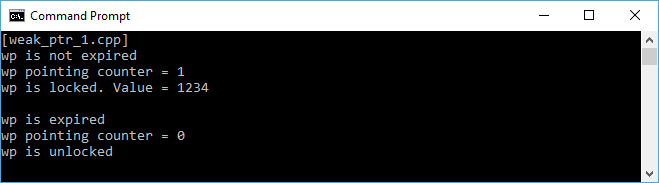At first, we instantiate `shared_ptr` and, as we discussed previously, the `weak_ptr` points to the object managed by `shared_ptr`. We then assign `wp` to the `shared_ptr` variable, `sp`. After we have a `weak_ptr` pointer, we then check its behavior. By calling the `expired()` method, we can figure out whether the referenced object was already deleted. And, since the `wp` variable is just constructed, it is not expired yet. The `weak_ptr` pointer also holds the value of the object counting by calling the `use_count()` method, as we used in `shared_ptr`. We then invoke the `locked()` method to create a `shared_ptr` that manages the referenced object and finds the value `weak_ptr` is pointing at. We now have a `shared_ptr` variable pointing to the address that holds the `1234` value.

We reset `sp` to `nullptr` afterward. Although we don't touch the `weak_ptr` pointer, it is also changed. As we can see from the console screenshot, now `wp` is expired since the object has been deleted. The counter also changes and becomes `0` since it points to nothing. Moreover, it is unlocked since the `shared_ptr` object has been deleted.

## Storing many different data types using tuples

We will get acquainted with tuples, an object that is able to hold a collection of elements, and each element can be of a different type. It is a new feature in C++11 and gives power to functional programming. The tuples will be most useful when creating a function that returns the value. Moreover, since functions don't change the global state in functional programming, we can return the tuples for all the values we need to change instead. Now, let's examine the following piece of code:

```    /* tuples_1.cpp */
#include <tuple>
#include <iostream>

using namespace std;

auto main() -> int
{
cout << "[tuples_1.cpp]" << endl;

// Initializing two Tuples
tuple<int, string, bool> t1(1, "Robert", true);
auto t2 = make_tuple(2, "Anna", false);

// Displaying t1 Tuple elements
cout << "t1 elements:" << endl;
cout << get<0>(t1) << endl;
cout << get<1>(t1) << endl;
cout << (get<2>(t1) == true ? "Male" : "Female") << endl;
cout << endl;

// Displaying t2 Tuple elements
cout << "t2 elements:" << endl;
cout << get<0>(t2) << endl;
cout << get<1>(t2) << endl;
cout << (get<2>(t2) == true ? "Male" : "Female") << endl;
cout << endl;

return 0;
}```

In the preceding code, we created two tuples, `t1` and `t2`, with different constructing techniques using `tuple<int, string, bool>` and `make_tuple`. However, these two different techniques will give the same result. Obviously, in the code, we access each element in tuples using `get<x>(y)`, where `x` is the index and `y` is the tuple object. And, with confidence, we will get the following result on the console: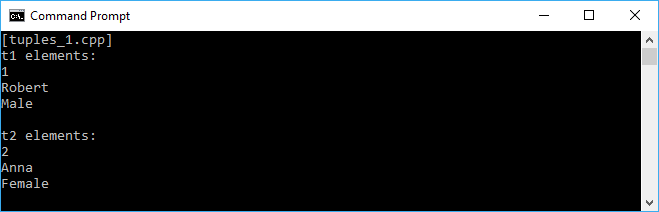### Unpacking tuples values

Another useful member that functions in the tuples classes is `tie()`, which is used to unpack a tuple into individual objects or create a tuple of `lvalue` references. Also, we have the `ignore` helper class in tuples, a placeholder to skip an element when unpacking a tuple is using `tie()`. Let's see the use of `tie()` and `ignore` in the following block of code:

```    /* tuples_2.cpp */
#include <tuple>
#include <iostream>

using namespace std;

auto main() -> int
{
cout << "[tuples_2.cpp]" << endl;

// Initializing two Tuples
tuple<int, string, bool> t1(1, "Robert", true);
auto t2 = make_tuple(2, "Anna", false);

int i;
string s;
bool b;

// Unpacking t1 Tuples
tie(i, s, b) = t1;
cout << "tie(i, s, b) = t1" << endl;
cout << "i = " << i << endl;
cout << "s = " << s << endl;
cout << "b = " << boolalpha << b << endl;
cout << endl;

// Unpacking t2 Tuples
tie(ignore, s, ignore) = t2;
cout << "tie(ignore, s, ignore) = t2" << endl;
cout << "new i = " << i << endl;
cout << "new s = " << s << endl;
cout << "new b = " << boolalpha << b << endl;
cout << endl;

return 0;
}```

In the preceding code, we have the same two tuples that `tuples_1.cpp` has. We want to unpack `t1` into variables `i`, `s`, and `b` respectively, using the `tie()` method. Then, we unpack `t2` to the `s` variable only, ignoring the `int` and `bool` data in `t2`. If we run the code, the output should be as follows: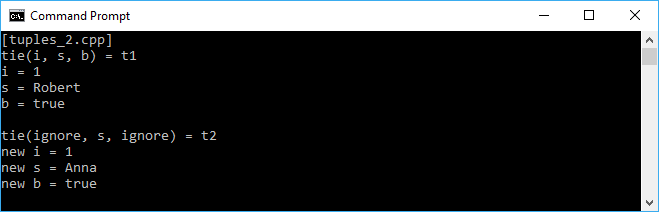### Returning a tuple value type

As we discussed earlier, we can maximize the use of tuples in functional programming when we want to write a function that returns multiple data. Let's take a look at the following block of code to know how to return the tuple and access the return value:

```    /* tuples_3.cpp */
#include <tuple>
#include <iostream>

using namespace std;

tuple<int, string, bool> GetData(int DataId)
{
if (DataId == 1)
return std::make_tuple(0, "Chloe", false);
else if (DataId == 2)
return std::make_tuple(1, "Bryan", true);
else
return std::make_tuple(2, "Zoey", false);
}

auto main() -> int
{
cout << "[tuples_3.cpp]" << endl;

auto name = GetData(1);
cout << "Details of Id 1" << endl;
cout << "ID = " << get<0>(name) << endl;
cout << "Name = " << get<1>(name) << endl;
cout << "Gender = " << (get<2>(name) == true ?
"Male" : "Female");
cout << endl << endl;

int i;
string s;
bool b;
tie(i, s, b) = GetData(2);
cout << "Details of Id 2" << endl;
cout << "ID = " << i << endl;
cout << "Name = " << s << endl;
cout << "Gender = " << (b == true ? "Male" : "Female");
cout << endl;

return 0;
}```

As we can see in the preceding code, we have a new function named `GetData()` returning a `Tuple` value. From that function, we will consume the data returning from it. We begin with creating the name variable and get the value from the `GetData()` function. We can also use the `tie()` method to unpack the tuple coming from the `GetData()` function, as we can see in the code when we access the data when ID = `2`. The output on the console should be like the following screenshot when we run the code: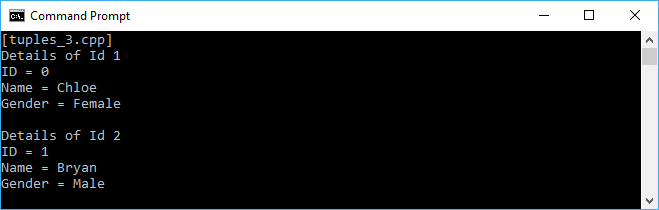## Summary

We have refreshed our experience in the C++ language by completing this chapter. Now we know that C++ is more modern, and it comes with numerous features that assist us in creating a better program. We can use the Standard Library to make our code efficient since we don't need to write too many redundant functions. We can use the Lambda expression to make our code tidy, easy to read, and easy to maintain. We can also use the smart pointer so we don't need to worry about memory management anymore. Moreover, as we are concerned about immutability in functional programming, we will discuss that deeper in the next chapter; the use of tuples can help us ensure that no global state is involved in our code.

In the next chapter, we will discuss First-Class and Pure Function, which is used to purify our class and ensure that no outside state is involved in the current function. As a result, it will avoid side effects in our functional code.

• ##### Wisnu Anggoro

Wisnu Anggoro is a Microsoft Certified Professional in C# programming and an experienced C/C++ developer. He has also authored the books Boost.Asio C++ Network Programming - Second Edition and Functional C# by Packt. He has been programming since he was in junior high school, which was about 20 years ago, and started developing computer applications using the BASIC programming language in the MS-DOS environment. He has solid experience in smart card programming, as well as desktop and web application programming, including designing, developing, and supporting the use of applications for SIM Card Operating System Porting, personalization, PC/SC communication, and other smart card applications that require the use of C# and C/C++.

He is currently a senior smart card software engineer at CIPTA, an Indonesian company that specializes in innovation and technology for smart cards. He can be reached through his email at [email protected]

Browse publications by this author

### Latest Reviews

(4 reviews total)